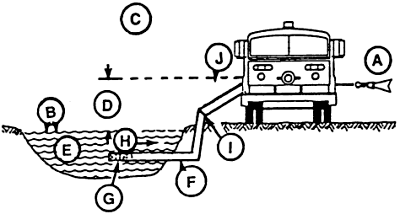# Factors Affecting Maximum Flow from Dry Hydrants and Dry Hydrant Sizing and Standardization

## Maximum Flow Factors### Maximum flow [A] is dependant on Maximum Usable Pressure (MUP)

Where:

• Atmospheric pressure [B]
• Elevation above sea level
• Atmospheric conditions [C]
• Static lift [D]
• Water vapor pressure [E]
• Water temperature
• Losses in Dry Hydrant
• Pipe and fittings [F]
• Diameter
• Length
• Interior Roughness
• Strainer
• Area of openings
• Diameter of pipe
• Loss in sudden reduction [I]
• Ration of DL and DS
• Loss in suction hose [J]
• Length
• Diameter

### Maximum Usable Pressure (MUP) = AP - SL - VP - MPP

Where:

• AP = atmospheric pressure in psi
• SL = static life in psi
• VP = vapor pressure in psi
• MPP = minimum pump pressure - pressure required at the suction of the pump